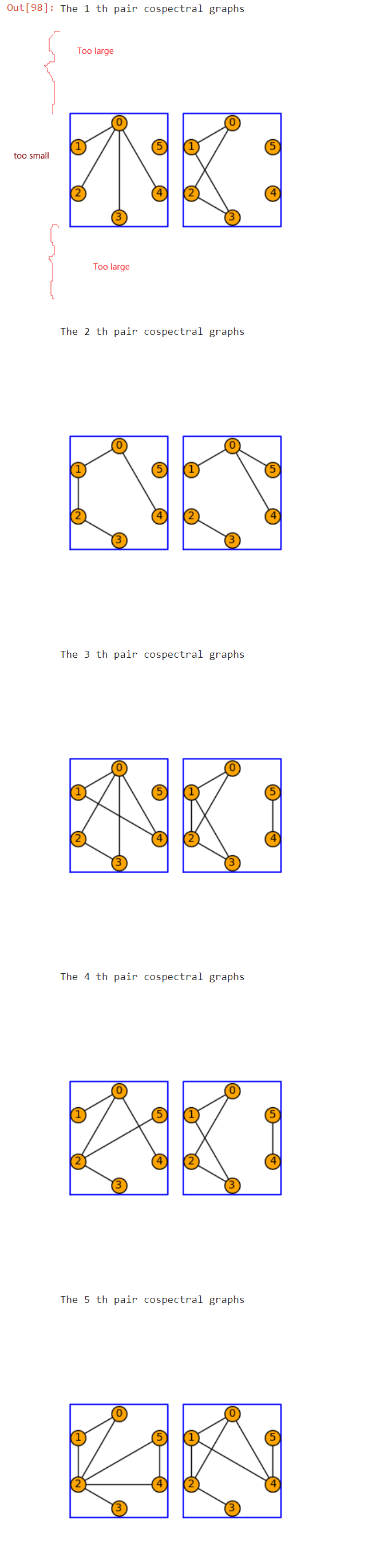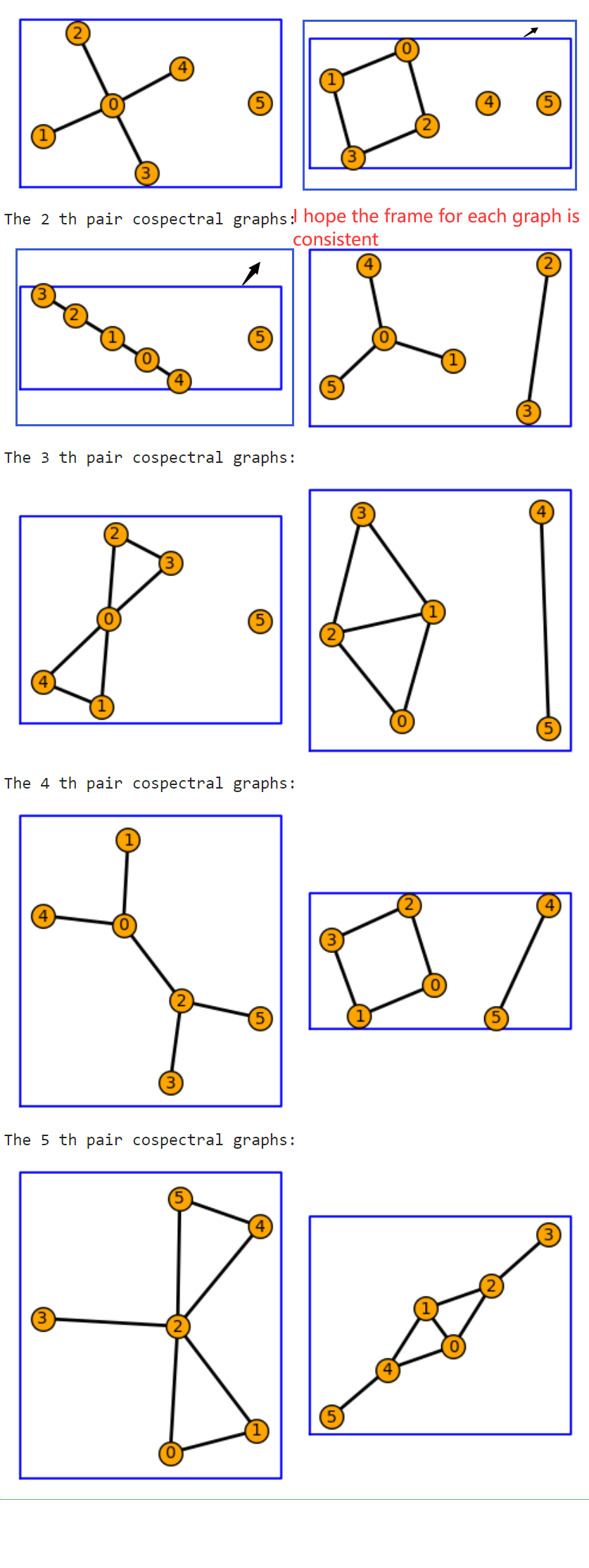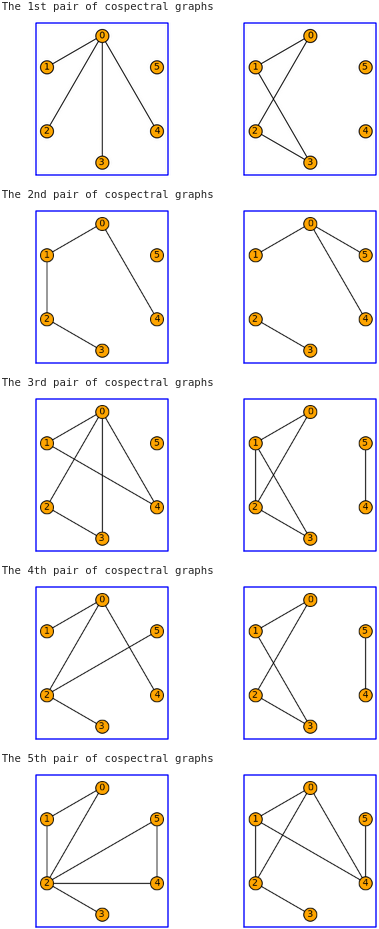# How to adjust the size of graphs in graphs_list.show_graphs

The following code is used to find some cospectral graphs. I want to show it in pairs with a for-loop. But each line of the graphs looks too small and there is too much space between each pair of graphs. I want to show them in a beautiful way.

g=graphs.cospectral_graphs(6)
l=len(g)
for i in range(l):
print("The",i+1, "th pair cospectral graphs")
graphs_list.show_graphs(g[i],vertex_size=200,figsize=100,vertex_labels=True,vertex_color='orange')I tried another method. I put a frame around each graph (like the blue border above). But the frame for each graph is not is inconsistent (see in the following picture). (The reason for putting a frame around each graph is to better distinguish the different graphs)

g=graphs.cospectral_graphs(6)
l=len(g)
for i in range(l):
G=[plot(g[i][j],graph_border=True,edge_thickness=2, vertex_size=200,vertex_labels=True,vertex_color='orange') for j in range(len(g[i]))]
print("The",i+1, "th pair cospectral graphs:")
graphics_array(G).show(figsize=5)edit retag close merge delete

1

This question relates to the presentation of the result computed by Sage, and depends on the interface you are using. Therefore, you should aim your question :

• to a Jupyter forum/mailing list if you use the Jupyter notebook

• to a LaTeX forum/mailing list if you aim to produce a PDF

• etc...

You may also work along the lines of graphics_array([[v.plot() for v in u] for u in graphs.cospectral_graphs(6)]) which leaves you entirely responsible for the formatting...

HTH

Sort by » oldest newest most voted

Not sure how to customize the graphics produced by graphs_list.show_graphs.

As you figured out, an alternative approach is to

• use graphics_array
• specify some options to the plot method of each graph

Here are some options that will hopefully work for you.

G = graphs.cospectral_graphs(6)
opt = dict(vertex_size=200, vertex_labels=True, vertex_color='orange',
graph_border=True, layout='circular', xmin=-1.5, xmax=1.5)
n_st = {1: 'st', 2: 'nd', 3: 'rd'}
n_th = lambda n: n_st[n % 10] if n % 10 in (1, 2, 3) and (n % 100) // 10  - 1 else 'th'
nth = lambda n: f'{n}{n_th(n)}'
for n, gg in enumerate(G, start=1):
print(f"The {nth(n)} pair of cospectral graphs")
graphics_array([g.plot(**opt) for g in gg]).show()more